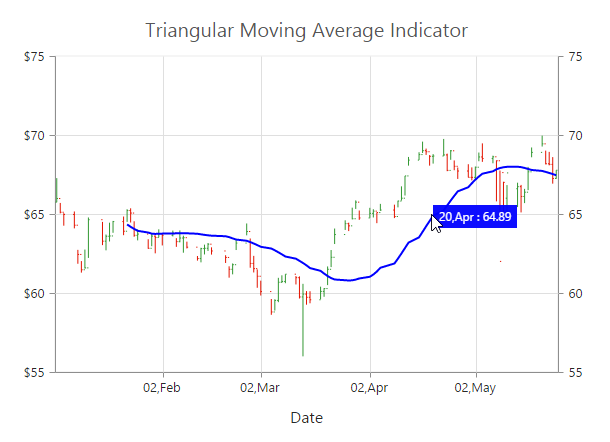# Technical Indicators

12 May 201716 minutes to read

EjChart control supports 10 types of technical indicators.

## Bind data to render the indicator

You can bind the series `dataSource` to the indicator by setting the specific series name to the indicator by using the `indicators.seriesName` property.

• JAVASCRIPT
• ``````"use strict";
//  ...
//Initializing Series
var series=[{
dataSource: chartData,
xName: "xDate",
high: "High",
low: "Low",
open: "Open",
close: "Close",
//Set name to series
name: 'Hilo',
//  ...
}];

//Initializing Indicators
var indicators= [{
//Set Hilo series dataSource to indicator using seriesName
seriesName: "Hilo",
//  ...
}];
//  ...
ReactDOM.render(
<EJ.Chart id="default_chart_sample_0"
series={series}
indicators={indicators}
>

</EJ.Chart>,
document.getElementById('chart')
);``````

Also, you can add data to the indicator directly by using the `dataSource` option of the indicator.

• JAVASCRIPT
• ``````"use strict";
//Initializing Indicators
var indicators= [{
dataSource: chartData,
xName: "xDate",
high: "High",
low: "Low",
open: "Open",
close: "Close",
//  ...
}];
//  ...
ReactDOM.render(
<EJ.Chart id="default_chart_sample_0"
indicators={indicators}
>

</EJ.Chart>,
document.getElementById('chart')
);``````

## Indicator Types

### Accumulation Distribution

To create an Accumulation Distribution indicator, set the `indicators.type` as “accumulationdistribution”. Accumulation Distribution require ‘volume’ field additionally with the `dataSource` to calculate the signal line.

• JAVASCRIPT
• ``````"use strict";
//  ...
//Initializing Series
var series=[{
name: "Hilo",
dataSource: chartData,
xName: "xDate",
high: "High",
low: "Low",
open: "Open",
close: "Close",
volume: "Volume",
//  ...
}];

//Initializing Indicators
var indicators= [{
seriesName: "Hilo",
//Set indicator type
type: "accumulationdistribution",
//  ...
}];
//  ...
ReactDOM.render(
<EJ.Chart id="default_chart_sample_0"
indicators={indicators}
series={series}
>

</EJ.Chart>,
document.getElementById('chart')
);``````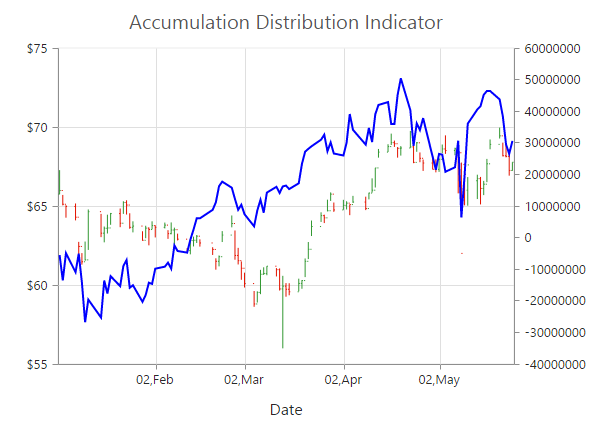### Average True Range (ATR)

You can create an ATR indicator by setting the `indicators.type` as “atr” in the `indicators`.

• JAVASCRIPT
• ``````"use strict";
//Initializing Indicators
var indicators= [{
//Set indicator type
type: "atr",
//  ...
}];
//  ...
ReactDOM.render(
<EJ.Chart id="default_chart_sample_0"
indicators={indicators}
>

</EJ.Chart>,
document.getElementById('chart')
);``````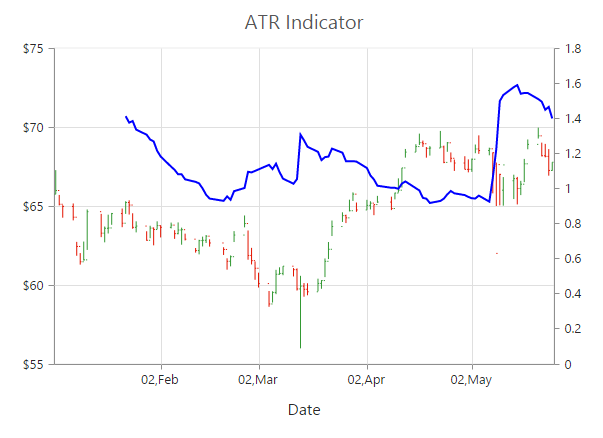### Bollinger Band

Bollinger Band indicator is created by setting the `indicators.type` as “bollingerband”. It contains three lines, namely upper band, lower band and signal line. Bollinger Band default value of the period is 14 and standardDeviations is 2.

• JAVASCRIPT
• ``````"use strict";
//Initializing Indicators
var indicators= [{
//Set indicator type
type: "bollingerband",
//  ...
}];
//  ...
ReactDOM.render(
<EJ.Chart id="default_chart_sample_0"
indicators={indicators}
>

</EJ.Chart>,
document.getElementById('chart')
);``````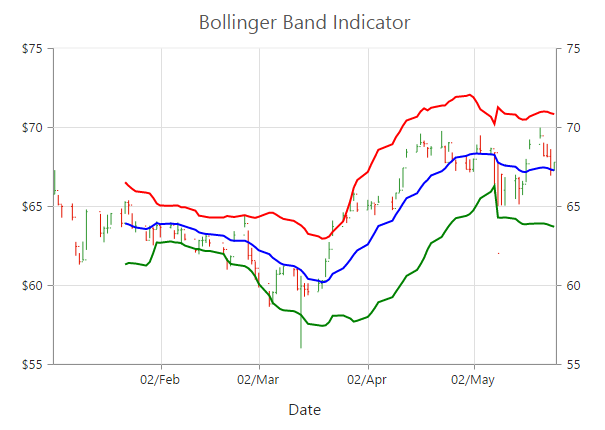### Exponential Moving Average (EMA)

To render an EMA indicator, you have to set the `indicators.type` as “ema”.

• JAVASCRIPT
• ``````"use strict";
//Initializing Indicators
var indicators= [{
//Set indicator type
type: "ema",
//  ...
}];
//  ...
ReactDOM.render(
<EJ.Chart id="default_chart_sample_0"
indicators={indicators}
>

</EJ.Chart>,
document.getElementById('chart')
);``````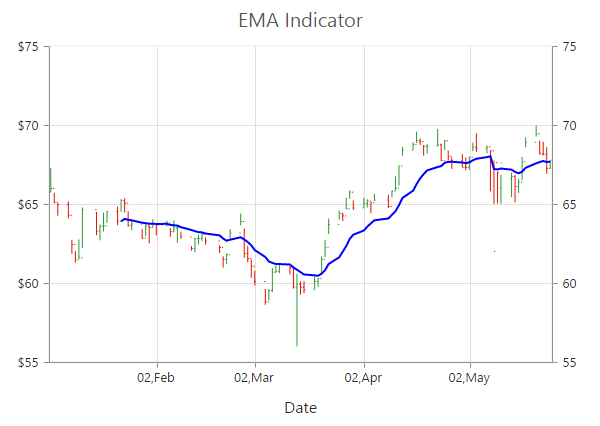### Momentum

Momentum Technical indicator is created by setting the `indicators.type` as “momentum”. The momentum indicator renders two lines, namely upper band and signal line. Upper band always rendered at the value 100 and the signal line is calculated based on the momentum of the data.

• JAVASCRIPT
• ``````"use strict";
//Initializing Indicators
var indicators= [{
//Set indicator type
type: "momentum",
//  ...
}];
//  ...
ReactDOM.render(
<EJ.Chart id="default_chart_sample_0"
indicators={indicators}
>

</EJ.Chart>,
document.getElementById('chart')
);``````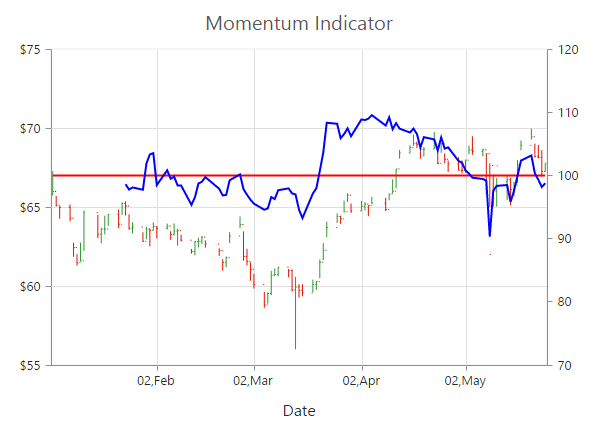### Moving Average Convergence Divergence (MACD)

To render an MACD indicator, you have to set the `indicators.type` as “macd”. MACD indicator contains MACD line, Signal line and Histogram column. Histogram is used to differentiate MACD and signal line.

• JAVASCRIPT
• ``````"use strict";
//Initializing Indicators
var indicators= [{
//Set indicator type
type: "macd",
//  ...
}];
//  ...
ReactDOM.render(
<EJ.Chart id="default_chart_sample_0"
indicators={indicators}
>

</EJ.Chart>,
document.getElementById('chart')
);``````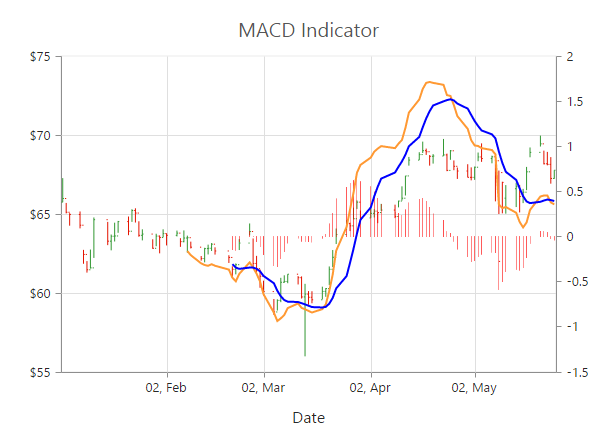#### macdType

By using the `macdType` enumeration property, you can change the MACD rendering as line, histogram or both.

• JAVASCRIPT
• ``````"use strict";
//Initializing Indicators
var indicators= [{
//Set indicator type
type: "macd",
//Set macd draw type
macdType: "histogram",
//  ...
}];
//  ...
ReactDOM.render(
<EJ.Chart id="default_chart_sample_0"
indicators={indicators}
>

</EJ.Chart>,
document.getElementById('chart')
);``````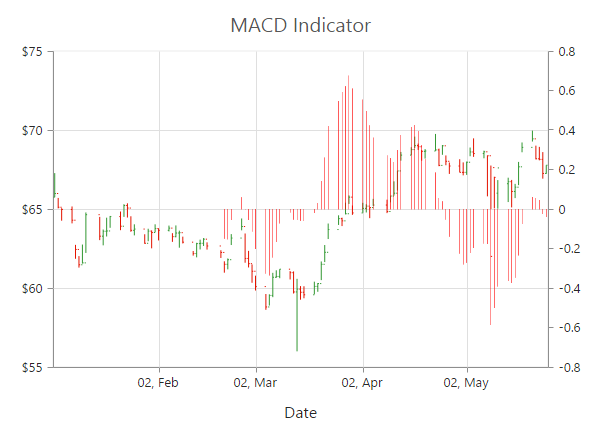### Relative Strength Index (RSI)

To render the RSI indicator, set the `indicators.type` as “rsi”. It contains three lines, namely upper band, lower band and signal line. Upper and lower band always render at value 70 and 30 respectively and signal line is calculated based on the RSI formula.

• JAVASCRIPT
• ``````"use strict";
//Initializing Indicators
var indicators= [{
//Set indicator type
type: "rsi",
//  ...
}];
//  ...
ReactDOM.render(
<EJ.Chart id="default_chart_sample_0"
indicators={indicators}
>

</EJ.Chart>,
document.getElementById('chart')
);``````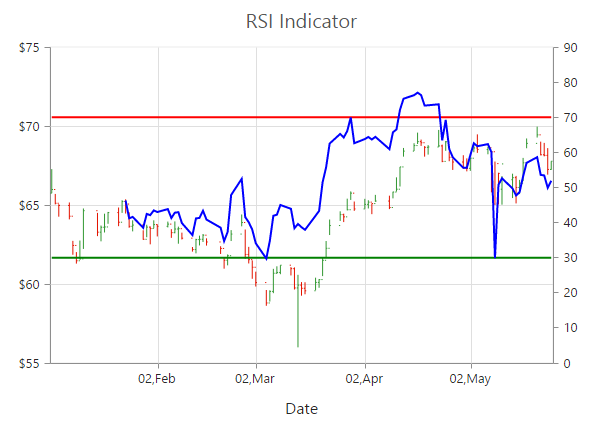### Simple Moving Average (SMA)

To render the SMA indicator, you should specify the `indicators.type` as “sma”.

• JAVASCRIPT
• ``````"use strict";
//Initializing Indicators
var indicators= [{
//Set indicator type
type: "sma",
//  ...
}];
//  ...
ReactDOM.render(
<EJ.Chart id="default_chart_sample_0"
indicators={indicators}
>

</EJ.Chart>,
document.getElementById('chart')
);``````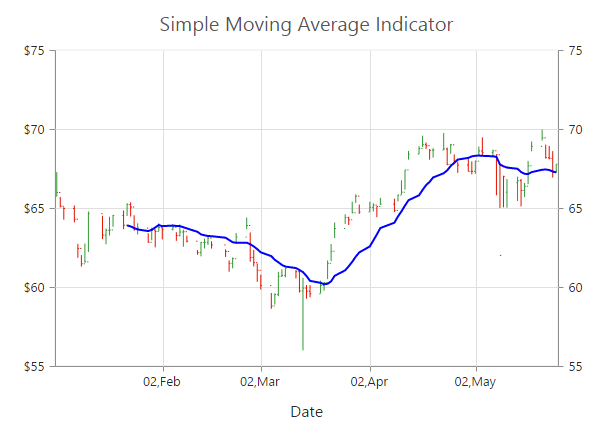### Stochastic

For the Stochastic indicator, you need to set the `indicators.type` as “stochastic”. The Stochastic indicator renders four lines namely, upper line, lower line, stochastic line and the signal line. Upper line always rendered at value 80 and the lower line is rendered at value 20. Stochastic and Signal Lines are calculated based on the stochastic formula.

• JAVASCRIPT
• ``````"use strict";
//Initializing Indicators
var indicators= [{
//Set indicator type
type: "stochastic",
//  ...
}];
//  ...
ReactDOM.render(
<EJ.Chart id="default_chart_sample_0"
indicators={indicators}
>

</EJ.Chart>,
document.getElementById('chart')
);``````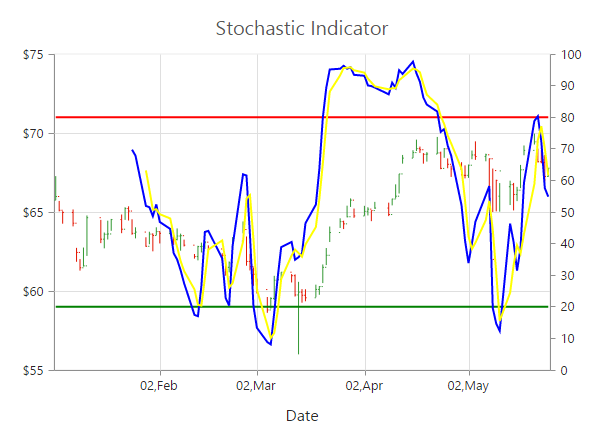### Triangular Moving Average (TMA)

To render the TMA indicator, you should specify the `indicators.type` as “tma”.

• JAVASCRIPT
• ``````"use strict";
//Initializing Indicators
var indicators= [{
//Set indicator type
type: "tma",
//  ...
}];
//  ...
ReactDOM.render(
<EJ.Chart id="default_chart_sample_0"
indicators={indicators}
>

</EJ.Chart>,
document.getElementById('chart')
);``````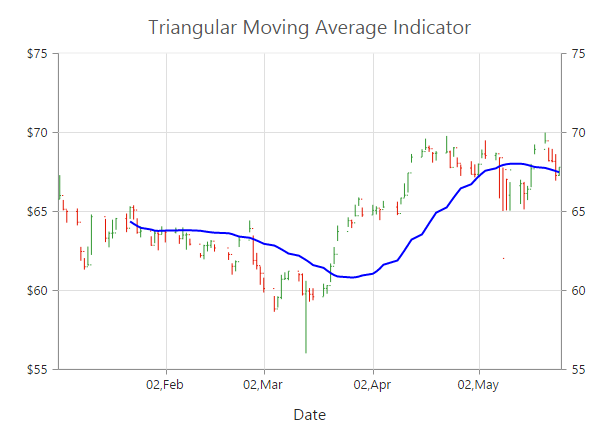## Enable Tooltip

To display the indicator tooltip, use `visible` option of the `indicators.tooltip`. Also, you can change and customize the tooltip color, border, format and font properties similar to the series tooltip.

• JAVASCRIPT
• ``````"use strict";
//Initializing Indicators
var indicators= [{
//  ...
tooltip: {
//Enable tooltip for indicator
visible: true
},
}];
//  ...
ReactDOM.render(
<EJ.Chart id="default_chart_sample_0"
indicators={indicators}
>

</EJ.Chart>,
document.getElementById('chart')
);``````